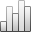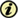More Statisticssince 05 February 2011 :
View(s): 258 (7 ULiège)
Fabienne Prosmans

### DERIVED LIMITS IN QUASI-ABELIAN CATEGORIES

Article#### Annexes

Mots-clés : non-abelian homological algebra, quasi-abelian categories, derived projective limits, homological methods for functional analysis

#### Abstract

In this paper, we study the derived functors of projective limit functors in quasi-abelian categories. First, we show that if is a quasi-abelian category with exact products, projective limit functors are right derivable and their derived functors are computable using a generalization of a construction of Roos. Next, we study index restriction and extension functors and link them trough the symbolic Hom-functor. If  is a functor between small categories and if E is a projective system indexed by , this allows us to give a condition for the derived projective limits of E and E J to be isomorphic. Note that this condition holds, if  and  are filtering and J is cofinal. Using the preceding results, we establish that the n-th left cohomological functor of the derived projective limit of a projective system indexed by  vanishes for n k, if the cofinality of  is strictly lower than the k-th infinite cardinal number. Finally, we consider the limits of pro-objects of a quasi-abelian category. From our study, it follows, in particular, that the derived projective limit of a filtering projective system depends only on the associated pro-object.

11991 AMS Mathematics Subject Classification : 18G50, 18A30,46M20